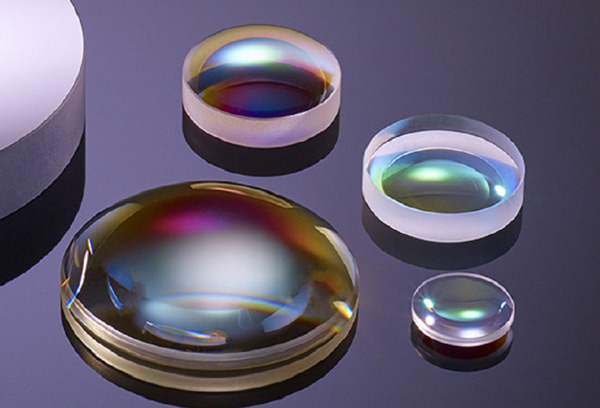# Best Choice of Spherical Lens

May. 24, 2022

According to the refraction of light and Snell's law, light will be refracted at the interface between the optical material and the air, resulting in a change in the direction of light propagation. Parallel rays of light converge to a point after passing through a double-convex lens and plano-convex lens. They diverge after passing through a double-concave lens and plano-concave lens. After the optical design, optical lenses converge and scatter the light, which plays the role of collimation, relay and imaging. Due to the different shapes of optical lenses, the light transmission effect, imaging effect and spherical aberration are also different.## Plano-convex lenses are suitable for the following situations:

1. Spherical lenses are used for infinity conjugate.

2. Object and image are located at different distances from spherical lenses.

3. The object is at infinity and the image is in focus.

4. Converging parallel light.

5. Turn the light of the point light source into a parallel light.

When the convex surface of a plano-convex lens is located at the maximum object distance, its asymmetry minimizes spherical aberration.

## Biconvex lenses are suitable for the following situations:

1. Spherical lenses are used for finite far conjugate.

2. The object distance is equal to the image distance.

3. Converging the light of a point source.

4. Forming or transferring an image in an optical system.

5. Doublet lenses.

Lenticular lenses have equal convex radius. Both surfaces of double convex lenses are symmetrical in both the horizontal and vertical directions. Therefore, lenticular lenses have less spherical aberration in the above case.

## Plano-concave lenses are suitable for the following situations:

1. Extend the focal length of optical systems.

2. Offset the aberration of optical systems.

3. Achromatic lenses.

In the optical systems, the concave surface of a plano-concave lens is generally toward the infinite conjugate. But when the plano-concave lens is in a high-energy laser, in order to avoid virtual focus, the plane of the plano-concave lens is oriented towards the infinite conjugate.

## Bi-concave lenses are suitable for the following situations:

The object distance and the image distance are close to the same infinite conjugate, and the incident rays are convergent rays.

CLZ Precision Optics provides you spherical lenses with custom ptical materials and sizes, such as N-BK7 biconvex lenses, fused silica biconvex lenses, N-BK7 plano-concave lenses and fused silica plano-convex lenses. In addition, we also manufacture custom achromatic lenses. Besides, optical coatings for custom optical components are available, according to specific requirements. Looking forward to your inquiry.